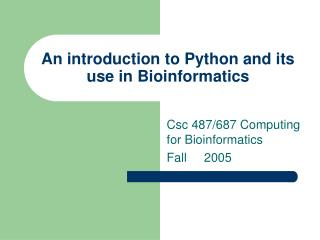DownloadDownload PresentationAn introduction to Python and its use in Bioinformatics

# An introduction to Python and its use in Bioinformatics

Télécharger la présentation## An introduction to Python and its use in Bioinformatics

- - - - - - - - - - - - - - - - - - - - - - - - - - - E N D - - - - - - - - - - - - - - - - - - - - - - - - - - -
##### Presentation Transcript

1. An introduction to Python and its use in Bioinformatics Csc 487/687 Computing for Bioinformatics Fall 2005

2. ifexpression: action Example: a1 = 'A‘; a2 = 'C'; match = 0; if (a1 == a2) : match+=1; if Statement

3. ifexpression: action 1 elif expression: action 2else : action 3 Example: a1 = 'A‘; a2 = 'C'; match = 0; gap = 0; if (a1 == a2) : match+=1; elif (a1 > a2): else: gap+=1; if-elif-else Statement

4. String operations

5. Lists

6. Dictionaries

7. for var in list: action Sets var to each item in list and performs action range() function generates lists of numbers: range (5) -> [0,1,2,3,4] Example mylist=[“hello”,”hi”,”hey”,”!”]; for i in mylist: print i for Statement Iteration 1 prints: hello Iteration 2 prints: hi Iteration 3 prints: hey Iteration 4 prints: !

8. while expression: action Example x = 0; while x != 3: x = x + 1 while Statement / 2 Infinite loop! Iteration 1: x=0+1=1 Iteration 2: x=1+1=2 Iteration 3: x=2+1=3 Iteration 4: don’t exec

9. Example: Amino Acid Search • Write a program to count the number of occurrences of an amino acid in a sequence. • The program should prompt the user for • A sequence of amino acids (seq) • The search amino acid (aa) • The program should display the number of times the search amino acid (aa) occurred in the sequence (seq)

10. Example: Amino Acid Search (2) #this program will calculate the number of occurrences of an amino acid in a sequence done=0 while (not done): sequence=raw_input("Please enter a sequence:"); aa=raw_input("Please enter the amino acid to look for:");

11. Example: Amino Acid Search (3) #compute the number of occurrences using for loop cnt=0 for i in sequence: if i == aa: cnt+=1 if cnt == 1: print "%s occurs in that sequence once" % aa; else: print "%s occurs in that sequence %d times" % (aa, cnt); answer=raw_input("try again? [yn]") if answer == "n" or answer == "N": done = 1

12. Programming Workshop #2 • Write a sliding window program to compute the %GC in a sequence of nucleotides. • The program should prompt the user for • The DNA sequence • The window size (assume the window increment is 1) • Inputs: sequence, window size • Outputs: nucleotide number, %GC for each window

13. Precise way to create a list Consists of an expression followed by a for clause, then zero or more for or if clauses Ex: >>> [str(round(355/113.0, i)) for i in range(1,6)] ['3.1', '3.14', '3.142', '3.1416', '3.14159'] Ex: >>> x = "acactgacct" >>> y = [int(i=='c' or i=='g') for i in x] >>> y Python List Comprehensions

14. To create a 2-D list L, with C columns and R rows initialized to 0: L = [[]] #empty 2-Dlist L = [[0 for col in range(C)] for row in range(R)] To assign the value 5 to the element at the 2nd row and 3rd column of L L = 5 Creating 2-D Lists

15. Zip – for parallel traversals • Visit multiple sequences in parallel • Ex: >>> L1 = [1,2,3] >>> L2 = [5,6,7] >>> zip(L1, L2) [(1,5), (2,6), (3,7)] • Ex: >>> for(x,y) in zip(L1, L2): … print x, y, '--', x+y

16. More on Zip • Zip more than two arguments and any type of sequence • Ex: >>> T1, T2, T3 = (1,2,3),(4,5,6),(7,8) >>> T3 (7,8) >>> zip(T1, T2, T3) ?

17. Dictionary Construction with zip • Ex: >>> keys = ['a', 'b', 'd'] >>> vals = [1.8, 2.5, -3.5] >>> hydro = dict(zip(keys,vals)) >>> hydro {'a': 1.8, 'b': 2.5, 'd': -3.5}

18. To open a file myfile = open('pathname', <mode>) modes: 'r' = read 'w' = write Ex: infile = open("D:\\Docs\\test.txt", 'r') Ex: outfile = open("out.txt", 'w') – in same directory File I/O

19. Common input file operations

20. Common output file operations

21. Extracting data from string – split • String.split([sep, [maxsplit]]) - Return a list of the words of the string s. • If the optional argument sep is absent or None, the words are separated by arbitrary strings of whitespace characters (space, tab, newline, return, formfeed). • If the argument sep is present and not None, it specifies a string to be used as the word separator. • The optional argument maxsplit defaults to 0. If it is nonzero, at most maxsplit number of splits occur, and the remainder of the string is returned as the final element of the list (thus, the list will have at most maxsplit+1 elements).

22. Split • Ex: >>> x = "a,b,c,d" >>> x.split(',') >>> x.split(',',2) • Ex: >>> y = "5 33 a 4" >>> y.split()

23. Functions • Function definition • def adder(a, b, c): return a+b+c • Function calls • adder(1, 2, 3) -> 6

24. Functions – Polymorphism >>>def fn2(c): … a = c * 3 … return a >>> print fn2(5) 15 >>> print fn2(1.5) 4.5 >>> print fn2([1,2,3]) [1,2,3,1,2,3,1,2,3] >>> print fn2("Hi") HiHiHi

25. Functions - Recursion def fn_Rec(x): if x == []: return fn_Rec(x[1:]) print x, y = [1,2,3,4] fn_Rec(y) >>> ?

26. Programming Workshop #3 • Write a program to prompt the user for a scoring matrix file name and read the data into a dictionary • ftp://ftp.ncbi.nih.gov/blast/matrices/